Courses

# Test: Introduction Of Coordination Compounds

## 25 Questions MCQ Test Chemistry Class 12 | Test: Introduction Of Coordination Compounds

Description
This mock test of Test: Introduction Of Coordination Compounds for Class 12 helps you for every Class 12 entrance exam. This contains 25 Multiple Choice Questions for Class 12 Test: Introduction Of Coordination Compounds (mcq) to study with solutions a complete question bank. The solved questions answers in this Test: Introduction Of Coordination Compounds quiz give you a good mix of easy questions and tough questions. Class 12 students definitely take this Test: Introduction Of Coordination Compounds exercise for a better result in the exam. You can find other Test: Introduction Of Coordination Compounds extra questions, long questions & short questions for Class 12 on EduRev as well by searching above.
QUESTION: 1

### Only One Option Correct Type Direction (Q. Nos. 1-10) This section contains 10 multiple choice questions. Each question has four choices (a), (b), (c) and (d), out of which ONLY ONE is correct. Q.  Primary and secondary valency of Pt in [Pt(en)2CI2] are

Solution:

In [Pt(en)2CI2] the charge on the complex ion is +2 and coordination number is 6. Hence, the primary and secondary valency are +2 and 6 respectively.

QUESTION: 2

Solution:
QUESTION: 3

### The two complexes PtCI4 . 2NH3 and PtCI4 . 2KCI do not give precipitate of AgCI when treate with AgNO3. The structures of these complexes are

Solution:

Pt(NH3)2CI4] is neutral complex and no ions are formed in aqueous solution.

K,(PtCl6] → 2K+ + [PtCI6]2-
It does not have Cl- ion toffive precipitate of AgCI.

QUESTION: 4

A cobaltamine has the formula CoCI3 . xNH3. This when reacted with AgNO3 solution, one third of the chloride is precipitated. It can have the structure

Solution:

Total chlorine molecules are 3 and one third means one chlorine molecule precipitates. So, one chlorine has to be outside the coordination sphere and that is in option C.

QUESTION: 5

Which is the incorrect ?

Solution:

Mohr's salt is a doub le salt and it will give test for Fe2+ ion. It dissociates into simple substances or ions completely when dissolved in water.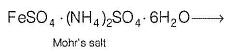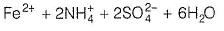QUESTION: 6

The spin only magnetic moment value (in Bohr magneton units) of Cr(CO)6 is

Solution:

Cr(24) = [Ar]3d54s1
Since (CO) is strong ligand, in Cr(CO)6 none of the electron is unpaired. Therefore ‘spin only’ magnetic moment is zero.

QUESTION: 7

The anion acetylacetonate (acac) forms chelate with Co3+, The ring of the chelate is

Solution:

The acetylacetonate (acac) forms six membered ring as follows: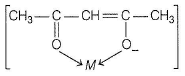QUESTION: 8

Oxidation states of iron in the complexes [Fe(H2O)5NO]2+ and [Fe(CN)5NO]2- are

Solution:

Explanation : NO in brown ring complex has +1 oxidation state.

Let oxidation state of Fe be x.

Since the overall charge on the coordination sphere, [Fe(H2O)5NO+]  is +2, the sum of oxidation states of all elements in it should be equal to +2.

Therefore, x+1=+2

or, x=+1

Hence, the oxidation state of iron in the brown ring complex is +1.

[Fe(H2O)5NO+]2- iron is assigned an oxidation state of 2+. The iron center has a diamagnetic low-spin d6 electron configuration, although a paramagnetic long-lived metastable state has been observed by EPR spectroscopy. The chemical reactions of sodium nitroprusside are mainly associated with the NO ligand.

QUESTION: 9

Complexes in which the oxidation number of a metal is zero is/are

Solution:

In [Ni(CO)4]
Let the oxidation state of Ni is x.
x + 4 x (0) = 0 (Carbonyl (CO) is a neutral ligand and have zero charge.)
x = 0
Hence, Ni have zero oxidation state.

QUESTION: 10

An example for bidentate and negatively charged ligand is

Solution:

CH3COCH2COCH3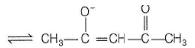It can ligate through two oxygen atoms.

*Multiple options can be correct
QUESTION: 11

One or More than One Options Correct Type

Direction (Q. Nos. 11-15) This section contains 5 multiple choice questions. Each question has four choices (a), (b), (c) and (d), out of which ONE or MORE THAN ONE are correct.

Q.

An example of double salt is/a

Solution:

Common alum is K2SO4.AI2(SO4)3.24H2O and carnallite is KCl.MgCl2.6H2O.

*Multiple options can be correct
QUESTION: 12

Type of bonding in K4 [Fe(CN)6] is/a

Solution:

The complex K4[Fe(CN)6] whose formula can be written like that of double salt. Fe(CN)2 . 4KCN, dissociates to give K+ and [Fe(CN)6]4- ions in the aqueous solution.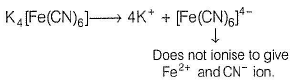QUESTION: 13

The effective atomic number of Fe in Fe(CO)5 is

Solution:

The answer is 36 because in coordination compound we use EAN method due to which inert group atomic number or noble gas atomic number is more stable.

*Multiple options can be correct
QUESTION: 14

Which of the following statements is/are correct?

Solution:

[Co(en)3]CI3 produces 4 ions in solution as follows :
[Co(en)3] CI3→ [Co(en)3]3+ + 3CI-

1. is correct as charge on the complex ion will be +1

2.  is incorrect as the complex will form 4 ions in solution

3. is correct as there is no charge on the complex

4. is also correct as cu+2 has blue color in solution

Hence A, C and D are correct.

*Multiple options can be correct
QUESTION: 15

A freshly prepared aqueous solution of Pd(NH3)2CI2 does not conduct electricity, it suggests that

Solution:

In the aqueous solution of Pd(NH3)2CI2, the atoms of chlorine I are in coordination sphere and the van't Hoff factor of the ] compound are unite.

QUESTION: 16

Comprehension Type

Direction (Q. Nos. 16 and 17) This section contains a paragraph, describing theory, experiments, data, etc. Two questions related to the paragraph have been given. Each question has only one correct answer among the four given options (a), (b), (c) and (d).

Passage

Complex compounds are addition compounds formed by the stoichiometric combination of two or more simple salts but do not decompose into constituent ions completely. The first such complex prepared by Tassaert is hexamine cobalt (III) chloride. Later many such compounds were prepared and their properties were studied. The chloramines complexes of cobalt (III) chromium (III) not only exhibit a spectrum of colours but also differ in the reactivity of their chlorides. Moreover, greater the number of ions produced by a complex in solution, greater is the electrical conductivity. This type of information was obtained for several series of complexes.

Q.

Coordination number of Co in CoCl3 . 5H2O is six. The volume of 0.1 N AgNO3 needed to precipitate the chlorine in 200 mL of 0.01 M solution of complex is

Solution:

Since, the oxidation number of Co is + 3 hence, the complex compound would be [CoCI . 5H2O]CI2.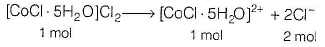Hence, 1 mole of [CoCI . 5H2O]CI2 gives 2 moles of Cl- ion.
Given, moles of [CoCI . 5H2O]CI2 = 200 mL x 0.01 M = 2 millimol
2 millimol of [CoCI . 5H2O]CI2 will give 4 millimol of Cl-.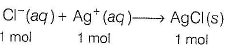Now, to neutralise 4 millimol of Cl-, 4 millimol of AgNO3 is required.
Let the volume o! AgNO3 = VmL
Millimoles of AgNO3 = 0.1 x V
0.1 x V = 4 millimol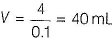QUESTION: 17

Complex compounds are addition compounds formed by the stoichiometric combination of two or more simple salts but do not decompose into constituent ions completely. The first such complex prepared by Tassaert is hexamine cobalt (III) chloride. Later many such compounds were prepared and their properties were studied. The chloramines complexes of cobalt (III) chromium (III) not only exhibit a spectrum of colours but also differ in the reactivity of their chlorides. Moreover, greater the number of ions produced by a complex in solution, greater is the electrical conductivity. This type of information was obtained for several series of complexes.

Q.

The number of ions per mole of the complex CoCI3 . 5NH3 in aqueous solution will be

Solution:

[Co(NH3)5CI]CI2→[Co(NH3)5CI]2++ 2Cl-
Hence, 3 ions are present for one mole of the complex in the solution.

QUESTION: 18

Matching List Type

Direction (Q. No. 18) Choices for the correct combination of elements from Column I and Column II are given as options (a), (b), (c) and (d), out of which one is correct.

Q.

'Match the Column I with Column II and mark the correct option from the codes given below.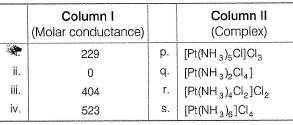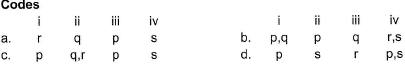Solution:

(i) → (r), (ii) → (q), (iii) → (p), (iv) → (s)

*Answer can only contain numeric values
QUESTION: 19

One Integer Value Correct Type

Direction (Q. Nos. 19-23) This section contains 5 questions. When worked out will result in an integer from 0 to 9 (both inclusive).

Q.

Formula of Mohr’s salt is FeSO4 . (NH4)2SO4 . xH2O. Here x value is

Solution:

Formula of Mohr’s salt is FeSO4 . (NH4)2SO4 . 6H2O . Here, x is 6

*Answer can only contain numeric values
QUESTION: 20

When AgNO3 solution is added in excess to 1 M solution of CoCI3 . xNH3, one mole of AgCI is formed. The value of x is

Solution:

AgNO3 solution is added in excess of 1 M solution of CoCI. xNH3.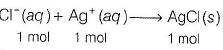The value of x is 4 as CoCI3 . 4NH3.

QUESTION: 21

The volume (in mL) of 0.1 M AgNO3 required for complex precipitation of chloride ions present in 30 mL of 0.01 M solution of [Cr(H2O)5Cl]Cl2, as silver chloride is close to

Solution:

The correct answer is Option D
Number of ionisable Cl− in [Cr(H2O)5Cl]Cl2 is 2.
∴ Millimoles of Cl− =30×0.01×2=0.6
∴ Millimoles of Ag+ required =0.6
Now, 0.6/V(in ml)   = 0.1
or, V=6 ml.

*Answer can only contain numeric values
QUESTION: 22

Oxidation state of cobalt in Co(CO)6 is

Solution:

ln Co(CO)6
Let the oxidation state of Co is x.
x + 6 x 0 = 0
[Carbonyl (CO) is a neutral ligand hence, zero charg]
x = 0
Hence, Co has zero oxidation state.

*Answer can only contain numeric values
QUESTION: 23

In the complex PtCl4 . 5NH3 if coordination number of platinum is 6, number of chloride ions precipitated by adding AgCI are

Solution:

Coordination number of platinum is 6.
∴ Number of chloride ions precipitated by adding AgCI are 3.

QUESTION: 24

Statement Type

Direction (Q. Nos. 24 and 25) This section is based on Statement I and Statement II. Select the correct answer from the codes given below.

Q.

Statement I : Oxidation state of Fe in Fe(CO)5 is zero.
Statement II : EAN of Fe in this complex is 36.

Solution:

Statement I is true because CO is a neutral ligand, Statement II is true because
EAN of Fe
= Atomic number of Fe + Electrons gained by coordination
= 26 + 2 x 5 = 36
But Statement II is not the correct explanation. Correct explanation is that oxidation state of Fe in Fe(CO)5 is zero because CO is a neutral ligand.

QUESTION: 25

Statement I : The oxidation num ber of platinum in Zeise’s salt is +4.

Statement II : Zeise’s salt is ionic complex.

Solution:

Statement I The oxidation number of Pt in Zeis e’s salt is +2.
Statement II Zeise’s salt is ionic complex with the formula K[PtCl3(C 2H4)].
Hence, statement II is correct but statment I is incorrect.